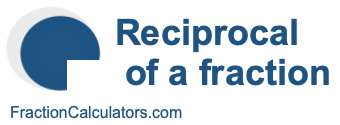Reciprocal of 3/5What is the reciprocal of 3/5? Here we will define reciprocal of 3/5, and show you how to calculate the reciprocal of 3/5 in fraction form and decimal form.

The reciprocal of 3/5 is a fraction or a number that when multiplied by 3/5 is equal to 1. To get the reciprocal of 3/5 based on that definition, we can make the following equation where "R" is the reciprocal of 3/5.

(3/5) × R = 1

When we solve for R, we get the answer to the reciprocal of 3/5 as a fraction as follows:

(3/5) × R = 1
R = 5/3
Reciprocal of 3/5 = 5/3

You can check that the answer is correct by confirming that 3/5 times the reciprocal of 3/5 is equal to 1, like this:

3/5 × 5/3 = 1

To find the decimal answer to the reciprocal of 3/5, you divide the numerator of the reciprocal by the demoninator of the reciprocal, like this:

5 ÷ 3 ≈ 1.666666666667
Reciprocal of 3/5 ≈ 1.666666666667

Tip: As you may have inferred from our tutorial above, you can quickly get the reciprocal of any fraction, such as 3/5, by switching the numerator and the denominator.

Reciprocal of a fraction
Do you need the reciprocal of another fraction? No problem! Please enter another fraction below.

Reciprocal of /

Reciprocal of 3/6
Here is the next fraction on our list that we have calculated the reciprocal of.